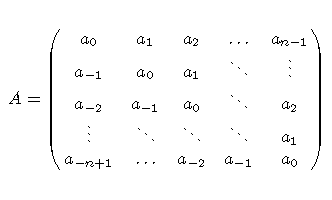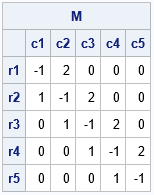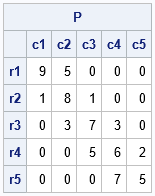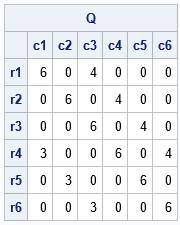Many useful matrices in applied math and statistics have a banded structure. Examples include diagonal matrices, tridiagonal matrices, banded matrices, and Toeplitz matrices. An example of an unsymmetric Toeplitz matrix is shown to the right. Notice that the matrix is constant along each diagonal, including sub- and superdiagonals.

Recently, I was performing some matrix computations in the SAS IML language that involved large, square, banded matrices. I wanted a fast way to specify the value for a sub- or superdiagonal for a large matrix. For small matrices, you can use the ROW and COL functions to assign values along a diagonal. However, that technique creates two matrices that are the same size as the original matrix. When the original matrix is large, such as 10,000 x 10,000, it is inefficient to create other large matrices.

This article shows a direct way to index the elements along a diagonal of a square matrix. In this article, "a diagonal" refers to any subdiagonal, superdiagonal, or the main diagonal.

### Direct indexing of tridiagonal elements

Tridiagonal matrices are encountered quite often, so let's start there.

In the SAS IML matrix language, elements are stores in row-major order. I have previously shown how to generate the indices of the elements of the main diagonal. It is not much harder to directly enumerate the indices on the first sub- and superdiagonal of an n x n matrix:

• The elements on the main diagonal have the indices 1, n+1, 2n+2, ..., n2. As a formula, these indices are 1 + k(n+1) for k=0,1,2,...,n-1.
• The elements on the first superdiagonal have the indices 2, n+3, 2n+4, .... As a formula, these indices are 2 + k(n+1) for k=0,1,2,...,n-2.
• The elements on the first subdiagonal have the indices n+1, 2n+2, 3n+3, .... As a formula, these indices are (n+1) + k(n+1) for k=0,1,2,...,n-2.

It is straightforward to convert these formulas to SAS IML function calls that return the indices of the various diagonals:

```proc iml; /* Functions that return the indices of the main diagonal, the subdiagonal, and the superdiagonal. */ start DiagIdx(n); k = 0:n-1; return 1 + k*(n+1); /* index diagonal elements */ finish; start SuperDiagIdx(n); k = 0:n-2; return 2 + k*(n+1); /* index super-diagonal elements */ finish; start SubDiagIdx(n); k = 0:n-2; return n+1 + k*(n+1); /* index sub-diagonal elements */ finish;   /* test the functions */ n = 5; M = j(n,n,0); /* assign constant value to each diagonal */ M[ DiagIdx(n) ] = -1; * set diag elements to -1; M[ SuperDiagIdx(n) ] = 2; * set super diag elements to +2; M[ SubDiagIdx(n) ] = 1; * set sub diag elements to +1; print M[c=('c1':'c5') r=('r1':'r5')];```The program assigns the value -1 to each element on the main diagonal. Elements on the superdiagonal are set to +2; Elements on the superdiagonal are set to +1.

The experienced programmer will realize that these functions return a vector of indices. Consequently, you can use the indices to assign a vector of values along the diagonals. That is, you can use the indices to assign n values to the main diagonal and n-1 values to the sub- and superdiagonals, as shown in the following example:

```P = j(n, n, 0); P[ DiagIdx(n) ] = T(9:5); /* assign 1x5 column vector */ P[ SuperDiagIdx(n) ] = {5,1,3,2}; /* assign 1x4 column vector */ P[ SubDiagIdx(n) ] = T(do(1,7,2)); /* assign 1x4 column vector */ print P[c=('c1':'c5') r=('r1':'r5')];```### Indices for any diagonal

The formulas in the previous section are similar and can be generalized. Let d indicate the diagonal you are referencing. Adopt the following conventions:

• Let d = 0 indicate the main diagonal.
• Let d > 0 indicate the superdiagonals. The first superdiagonal corresponds to d=1, the second superdiagonal corresponds to d=2, and so forth.
• Let d < 0 indicate the subdiagonals. The first subdiagonal corresponds to d=-1, the second subdiagonal corresponds to d=-2, and so forth.

The formulas require that you know the index of the first element on each diagonal. For d ≥ 0, the first index of the d_th superdiagonal is d+1. For d < 0, the first index of the |d|_th subdiagonal is n*|d| + 1. Therefore, you don't need the previous three functions. You only need one generalized function, which enables you to return the indices for any sub- or superdiagonal. The following function returns the indices of any subdiagonal (d < 0), main diagonal (d = 0), and superdiagonal (d > 0):

```start DiagIdx(n, d=0); if abs(d)>=n then return( . ); /* invalid index */ k = 0:n-abs(d)-1; if d>=0 then firstIdx = d+1; else firstIdx = abs(d)*n + 1; return firstIdx + k*(n+1); finish;   n = 6; Q = j(n, n, 0); Q[ DiagIdx(n) ] = 6; /* assign main diag */ Q[ DiagIdx(n, 2) ] = 4; /* assign 2nd super diag */ Q[ DiagIdx(n, -3) ] = 3; /* assign 3rd sub diag */ print Q[c=('c1':'c6') r=('r1':'r6')];```The example references the main diagonal, the second superdiagonal (elements set to +4), and the third subdiagonal (elements set to 3).

### Extract values of any diagonal

The previous examples have shown how to set the values along a diagonal. But you can use the same DiagIdx function to get the values along a diagonal. For example, the following statement gets the second superdiagonal for a square matrix named Q:

`d1 = Q[ DiagIdx(ncol(Q), 2) ]; /* get the 2nd superdiagonal of Q */`

### Summary

This article shows that you can directly generate the indices for the diagonal elements of a square matrix. (The formulas for a rectangular matrix are similar.) This is useful when computing with very large, banded matrices because you can efficiently assign the value of the matrix diagonals.

Share